# Natural Convection and Thermal Radiation Influence on Nanofluids in a Cubical Cavity

Natural Convection and Thermal Radiation Influence on Nanofluids in a Cubical Cavity

Lahcen El Moutaouakil Mohammed Boukendil* Zaki Zrikem Abdelhalim Abdelbaki

LMFE, Department of Physics, Cadi Ayyad University, Faculty of Sciences Semlalia, B.P. 2390 Marrakech, Morocco

Corresponding Author Email:
m.boukendil@uca.ac.ma
Page:
59-68
|
DOI:
https://doi.org/10.18280/ijht.380107
20 October 2019
|
Accepted:
14 January 2020
|
Published:
31 March 2020
| Citation

OPEN ACCESS

Abstract:

This study investigates the effect of thermal radiation on natural convection of several water-based nanofluids H2O-(Cu, Al2O3, Ag, TiO2) in a partially heated cubical cavity where the left vertical side is heated by three identical and parallel elements. The right vertical side is totally cooled, and the other ones are kept adiabatic. A developed code based on the finite volume method and the Rosseland approximation is used to solve the governing equations. Calculations were performed for three inclination angles of the rectangular heating elements 0°, 45° and 90°. The effect of governing parameters, namely, Rayleigh number, solid volume fraction, radiation parameter, dimensionless spacing of the three heating elements, their aspect ratio and different type of nanoparticles on the velocity contours, isotherms as well as local and average Nusselt number were considered. The results indicate that the inclination angle has a considerable effect on the dynamic and thermal fields, but its effect on the average heat transfer is insignificant. The total Nusselt number increases with the volume fraction of nanoparticles, the radiation parameter and the aspect ratio of the heated elements. The numerical results also revealed that the Cu and Ag-water nanofluid offer a better heat exchange.

Keywords:

heating elements, nanofluids, orientation, radiation effect, three-dimensional numerical simulation

1. Introduction

Heat transfer is the basis of many industrial processes that are present in everyday life. Convective heat transfer can be enhanced in several ways. One possibility is the thermal conductivity of traditional fluids (water, oil, ethylene glycol mixture….) which is the first limitations of thermal system performance. To overcome this disadvantage, there is a strong motivation to develop heat transfer with significantly higher thermal conductivities: that of nanofluids .

Numerical studies dedicated to natural convection of nanofluids in two-dimensional (2D) partially heated cavities are numerous. Many of them are cited in the detailed review by Haddad et al. . Oztop and Abu-Nada  numerically studied natural convection in a partially heated rectangular cavity considering different types of nanoparticles. They found that copper nanoparticles offer a better heat exchange than oxides Al2O3 and TiO2. It has also been found that the average convective Nusselt number increases with the size of the heating element and with the volume fraction of the nanoparticles. Similar remarks were reported by Hosseini et al.  using nanoparticles Cu, Ag, Al2O3 and TiO2 in a partially heated inclined square cavity. The effect due to the type of nanoparticles on heat transfer has been further highlighted by Bhuiyana et al.  considering a square cavity partially heated from its bottom wall. The transition to chaotic natural convection of Cu-water nanofluid inside an inclined square cavity is numerically investigated by Boudjeniba et al. . The enclosure is heated from one part of the side and cooled through two other opposite half sides. It was found that the presence of suspended nanoparticles inside the base fluid causes significantly the delay of this transition. Cho  has performed a numerical study into the heat transfer performance and entropy generation of natural convection in a partially-heated square cavity filled with Al2O3-water nanofluid. Their results indicate the increase of the average Nusselt number and the decrease of the total entropy with the volume fraction of the Al2O3 nanoparticles. Sheremet et al.  simulated the natural convection of a nanofluid in a partially heated porous cavity with a left isothermal wavy wall. The results indicate that the position of the hot element is decisive for the heat transfer in the cavity. Magnetohydrodynamic (MHD) natural convection of Cu-water nanofluid is analyzed by Sheremet et al.  considering an inclined wavy enclosure in the presence of an isothermal corner heater. The obtained results show that an insertion of nanoparticles leads to an attenuation of convective flow while the average Nusselt number increases with the solid volume fraction of nanoparticles. Mansour et al.  focused on the entropy generation and MHD natural convection in a porous cavity filled with the Cu-Al2O3-water hybrid nanofluid. An isothermal heat source is located on the upper and lower sides of the cavity and a heat sink are located on its sidewalls. It is noticed that the highest heat transfer is achieved using copper nanoparticles and that the entropy generated increases with the volume fraction of the nanoparticles. Wang et al.  studied natural convection in a partially heated enclosure filled with nanofluids having temperature-dependent properties. The obtained results show an optimal nanoparticles volume fraction at which the heat transfer performance has a peak. Ul Haq et al.  have analyzed the MHD natural convection inside a C-shape cavity filled with the water based SWCNTs (Carbon Nanotubes). The enclosure is partially heated by its left vertical wall. It is observed that increasing in nanoparticles volume fraction up to 20 % does not have significant impact on the flow field and temperature distribution. Sheremet and Pop  have investigated numerically free convection of nanofluid in a tilted porous cavity with a local isothermal heater. The obtained results indicate that the heat transfer improves and convective flow reduces when the distance between the heater and the cold vertical wall decreases. Guestal et al.  carried out a numerical study on heat transfer by natural convection of two nanofluids in a partially heated horizontal cylindrical enclosure. The Nusselt number was correlated in terms of the Rayleigh number, the heated length and the nanoparticles volume fraction. Ul Haq and Aman  studied the natural convection of the CuO-H2O nanofluid in a partially heated trapezoidal cavity with a square obstacle placed in its center. The results indicate that the convective heat transfer decreases by increasing the volume fraction of the nanoparticles and the size of the heating element.

The convection heat transfer of nanofluids has been studied in tridimensional (3D) structures. Wang et al.  investigated the effects of temperature-dependent properties on natural convection of Al2O3-H2O nanofluid in a partially heated enclosure. Selimefendigil and Öztop  investigated the MHD natural convection and the heat transfer characteristics in a 3D trapezoidal cavity filled with CuO-H2O nanofluid. Entropy generation analysis of water based Carbon Nanotubes nanofluid 3D flow over a inclined nonlinear stretching sheet embedded in porous media have been studied by Jain and Gupta . Aun et al.  performed an experiment to investigate the heat transfer characteristics by using low concentration of diamond water nanofluid in loop heat Pipe.

There are several specific problems of scientific and technological interest in which heat transfer via radiation and convection occurs in optically thick liquids, such as the flow of the earth mantle , the flow of oxide melts during crystal growth , and the processing of molten glass . So, recently, the focus shifted to coupling between natural convection-radiation in nanofluids which absorb and emit radiant energy. Karimipour  used the discrete ordinate method to consider the absorption and scattering coefficients of nanoparticles Al2O3 suspended in water as the base fluid in an inclined differentially heated tall cavity. The obtained results show a positive effect of the wave length on the radiation and the global heat flux through the cavity. Ghalambaz et al.  studied the influence of the viscous dissipation and radiation effects on the natural convection heat transfer in a square cavity filled with porous media saturated with a nanofluid. Due to viscous dissipation effect, their results show that the Nusselt numbers at the hot and cold walls are not equal. It is also found that the overall radiation effect increases the heat transfer.

This literature review shows that the natural flows of nanofluids in 2D and 3D cavities have been well studied. However, it was noted that the previous three-dimensional studies did not take into account the thermal radiation in the nanofluids which, according to the two-dimensional cited ones, seems to have a non-negligible effect on heat exchange in the cavities. To complete the existing works, the main objective of this paper is to analyze numerically the effect of thermal radiation and the inclination of three identical heated elements on the natural convection of nanofluids in a partially heated cubical cavity. The solution is obtained numerically using an in-house code based on well-known Finite Volume Method and the Rosseland approximation. For each inclination angle ($\gamma=0^{\circ}, 45^{\circ}$ and $90^{\circ}$) of the three elements, this study is conducted by analyzing the effects of the Rayleigh number ($10^{3} \leq R a \leq 10^{6}$), the volume fraction ($0 \leq \phi \leq 0.1$) and the nature of nanoparticles (Ag, Al2O3, Cu, TiO2), the radiation number ($0 \leq R d \leq 0.5$), the spacing D between the heated elements and their aspect ratio A=b/a (see Figure 1) on the flow structure and on the total heat transfer in the cavity. Coupling between natural convection of nanofluids and thermal radiation phenomena have many applications in electronic cooling. So, controlling the thermal behavior of the studied configurations is very important in engineering.

2. Studied Configurations

The studied geometries are shown in Figure $1 .$ It is a cubical cavity $(L=W=H)$ filled with several $\left(C u, A l_{2} O_{3}, A g, T i O_{2}\right)$ water-based nanofluids. On the plane $X=1$ are three identical and parallel elements, maintained at a high temperature $T_{H}$ These rectangular elements are either vertical (case I: $\gamma=0^{\circ}$ ), inclined at an angle $\gamma=45^{\circ}$ (case II) or horizontal (case III: $\left.\gamma=90^{\circ}\right) .$ The center of the second heating element coincides with that of the heated wall and is at an adjustable distance $D$ from the centers of the first and third ones. The wall $X=0$ is maintained at a cold temperature $T_{C}=T_{H}-\Delta T$. The remaining portion of the heated side and other walls are insulated. The thermophysical properties of the water and the used nanoparticles are given in Table 1. Those of the nanofluid can be estimated using the existing relations for two-phase mixture (Table 2). The density variation in the buoyancy term is estimated by using the Boussinesq approximation. It also assumed that the base fluid and nanoparticles are in thermodynamic equilibrium and that they flow at the same velocity. Nanofluid is supposed to be Newtonian, incompressible, homogeneous, isotropic and optically thick.

The conservation equations of mass, momentum and energy are:

$\frac{\partial U_{i}}{\partial X_{i}}=0$     (1)

\begin{aligned} \frac{D U_{i}}{D \tau}=& \frac{\rho_{f}}{\rho_{n f}}\left[-\frac{\partial P}{\partial X_{i}}+\frac{\mu_{n f}}{\mu_{f}} \operatorname{Pr}_{f} \frac{\partial^{2} U_{i}}{\partial X_{i}^{2}}\right.\\ &\left.+\delta_{i 3} \frac{(\rho \beta)_{n f}}{(\rho \beta)_{f}} \operatorname{RaPr}_{f} \theta\right] \end{aligned}     (2)

$\frac{D \theta}{D \tau}=\frac{\left(\rho C_{p}\right)_{f}}{\left(\rho C_{p}\right)_{n f}} \frac{k_{n f}}{k_{f}}\left(1+\frac{4}{3} R d\left[\frac{k_{n f}}{k_{f}}\right]^{-1}\right) \Delta \theta$      (3)

To obtain the non-dimensional governing Eqns. (1-3), the following dimensionless variables are used:

$X_{i}=\frac{x_{i}}{L}, U_{i}=\frac{u_{i} L}{\alpha_{f}}, \theta=\frac{T-T_{C}}{\Delta T}$     (4)

The dimensionless numbers in Eqns. (1-3) are given by:

$\operatorname{Pr}_{f}=\frac{v_{f}}{\alpha_{f}}, R a=\frac{g \beta \Delta T}{(v \alpha)_{f}} L^{3}, R d=\frac{4 \sigma T_{c}^{3}}{k_{f} \beta_{R}}$     (5)

Using the Rosseland approximation for thermal radiation and applying to optically thick media, the radiative heat flux is given by Raptis et al.  and Mahmoud :

$q_{r}=-\frac{4 \sigma}{3 \beta_{R}} \nabla T^{4} \approx-\frac{16 T_{C}^{3} \sigma}{3 \beta_{R}} \nabla T$      (6)

The boundary conditions for the governing equations are:

$U_{i}=0$ on all solid walls. $\theta=0$ for $X=0 . \theta=1$ on the heating elements and $\frac{\partial \theta}{\partial n}=0$ on the rest of the hot wall and on the remaining walls. $n$ is the direction perpendicular to the wall.

Local and average total Nusselt numbers are calculated using the following expressions:

$N u_{L}=\frac{k_{n f}}{k_{f}}\left(1+\frac{4}{3} R d\left[\frac{k_{n f}}{k_{f}}\right]^{-1}\right) \frac{\partial \theta}{\partial n}, N u=\iint N u_{L} d S$     (7)

## 1.png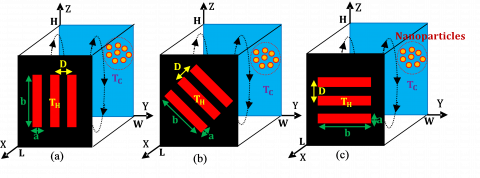Figure 1. (a) Case I: $\gamma=0^{\circ},$ (b) Case II: $\gamma=45^{\circ}$, (c) Case III: $\gamma=90^{\circ}$

Table 1. Thermophysical properties of water and nanoparticles

 Properties $H_{2} O$ $C u$ $A g$ $A l_{2} O_{3}$ $T i O_{2}$ $p$ 997.1 8933 10500 3970 4250 $k$ 0.613 400 418 40 8.9538 $C_{p}$ 4179 385 230 765 686.2 $\beta$ 21 1.67 1.65 0.85 0.9

Table 2. Thermophysical properties of nanofluid

 Nanofluid $\frac{\mu_{n f}}{\mu_{f}}$ $(1-\varphi)^{-2.5}$ $\frac{\rho_{n f}}{\rho_{f}}$ $1-\varphi+\varphi \frac{\rho_{s}}{\rho_{f}}$ $\frac{\left(\rho C_{P}\right)_{n f}}{\left(\rho C_{P}\right)_{f}}$ $1-\varphi+\varphi \frac{\rho_{s}}{\rho_{f}} \frac{C_{P n f}}{C_{P f}}$ $\frac{\beta_{n f}}{\beta_{f}}$ $\frac{1}{1+\frac{1-\varphi}{\varphi} \frac{\rho_{f}}{\rho_{s}}} \frac{\beta_{s}}{\beta_{f}}+\frac{1}{1+\frac{\varphi}{1-\varphi} \frac{\rho_{s}}{\rho_{f}}}$ $\frac{k_{n f}}{k_{f}}$ $\frac{\frac{k_{s}}{k_{f}}+2-2 \varphi\left(1-\frac{k_{s}}{k_{f}}\right)}{\frac{k_{s}}{k_{f}}+2+\varphi\left(1-\frac{k_{s}}{k_{f}}\right)}$
3. Numerical Procedure and Validation

To integrate Eqns. (1-3), the finite volume method was used. A third order Quick interpolation scheme is employed to minimize the numerical diffusion for advective terms. The resolution algorithm is based on the coupler of SIMPLE pressure equations. Various mesh sizes $\left(32^{3}, 52^{3}, 72^{3} \text { and } 92^{3}\right)$ have been tested in order to guarantee the grid-independency of the present solution. The results show that a grid of $72^{3}$ in the three directions $x, y$ and $z$ ensures a grid-independent solution.A refinement of the mesh at $92^{3}$ led to a high computation time, but it involves only weak deviations not exceeding $1.02 \%$ and $0.2 \%$ in terms of $W_{\text {Max}}$ and $N u,$ respectively for the extreme case of $R a=10^{6}, \gamma=45^{\circ}, \phi=0.1$ and $R d=0.5$.

In the presence of nanoparticles, the numerical code was verified by comparing isotherms (Figure 2) and average Nusselt number (Table 3) obtained for different combinations of governing parameters ($R a, \phi$) in a differentially heated cubical cavity with the results of Ravnik et al. . The qualitative tests giving the isotherms on the vertical median plane of the cavity show a great similarity between the two results. As it can be seen (Table 3), the maximum deviations recorded by comparing Nu numbers (quantitative tests) are less than 2.6 %.

## 2.png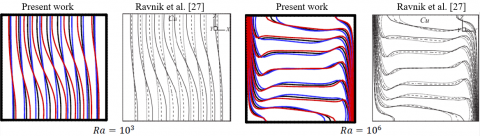Figure 2. Temperature contours on the central plane $Y=0.5$ for natural convection in a differentially heated cubical cavity with $C u-H_{2} O$ at different $R a$ numbers: $\phi=0$ (red), 0.1 (black) et 0.2 (blue)

Table 3. Validation in terms of Nusselt number for different combinations ($R a, \phi$)

 Ra Cu-H2O Al2O3-H2O  Present work  Present work $\phi=0$ 0.1 0.2 0 0.1 0.2 0.1 0.2 0.1 0.2 103 1.071 1.363 1.758 1.070 1.360 1.756 1.345 1.718 1.345 1.718 104 2.078 2.237 2.381 2.076 2.202 2.319 2.168 2.244 2.164 2.241 105 4.510 4.946 5.278 4.519 4.895 5.150 4.806 4.968 4.814 4.973 106 9.032 10.08 10.98 9.032 9.988 10.752 9.817 10.39 9.829 10.409
4. Results and Discussion

In the following text, the results are reported for a typical case of a $C u$ -water nanofluid with $P r=6.2$ and solid volume fraction $\phi=0.1$ (or 0 for pure water), the aspect ratio of the heating elements $A=4,$ the distance between them $D=1 / 6$ and radiation parameter $R d=0.5(0$ in absence of radiation). Otherwise, the values of non-dimensional parameters will be stated.

4.1 Ra number effect: $\boldsymbol{\phi}=\mathbf{0}, \boldsymbol{R} \boldsymbol{d}=\mathbf{0}$

Figure 3 shows the isotherms in the cubical cavity for different $R a$ number, $\phi=0$ (pure water), $R d=0$ (pure natural convection) and $\gamma=45^{\circ}$ (case II). The figure shows that the $R a$ number effect is considerable on the temperature field in the cavity. In its central part, the isotherms that are vertical and parallel to the active walls for $R a=10^{3}$ become horizontal (stratified) for $R a=10^{6} .$ In other words, at high Ra numbers, the upper part of the cavity is heated due to the buoyancy effect. This transition from the conductive to the convective regime is progressively accomplished. Indeed, for $R a=10^{4},$ it can be seen that the isothermal surfaces are inclined by about $45^{\circ}$ in the central zone of the cavity. For high $R a=10^{4},$ it can be seen that the isothermal surfaces are inclined by about $45^{\circ}$ in the central zone of the cavity. For high Ra numbers, it is seen that the isotherms are more closely packed at the bottom of the hot elements and at the top of the cold wall indicating an improvement of the heat transfers in the cavity. By moving away from the heated wall, the threedimensional aspect of the flow diminishes. In fact, in the vicinity of the cold wall, it is noticed that the isothermal surfaces are almost parallel to the $Y$ coordinate especially for reduced $R a$ numbers. However, it is clear that the fluid temperature varies in the three directions $(\mathrm{x}, \mathrm{y} \text { and } \mathrm{z}$ ) near the partially heated wall.

## 3.pngFigure 3. Isotherms for different Ra number: $\gamma=45^{\circ}, \phi=0$ and $R d=0$

## 4.png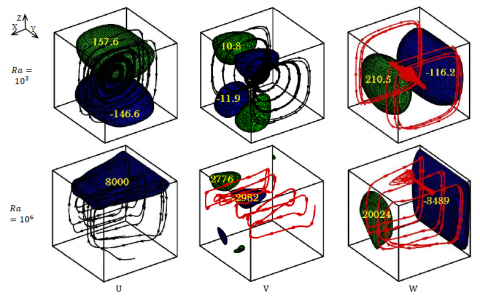Figure 4. Velocity contours for $\phi=0, R d=0$ and different Rayleigh numbers: (green) positive values, (blue) negative ones

In the absence of the thermal radiation $(R d=0),$ the results for the pure water $(\phi=0)$ are also shown (Figure 4 ) in terms of velocity contours obtained for $R a=10^{3}$ and $10^{6} .$ The general form of the three-dimensional flow is displayed by the streamlines illustrated in the same figure. For $R a=10^{3},$ it can be seen that the velocity components $U$ and $W$ constitute the main flow, in the vicinity of the horizontal passive $(Z=0$ and $Z=1$ ) and the active walls $(X=0 \text { and } X=1),$ respectively, suggesting that the fluid flow is characterized by a major clockwise circulation cell occupying the most of the cavity. Additionally, due the weakness of the buoyancy force, the U and W contours have almost similar shapes suggesting that the fluid flow is characterized by a major clockwise circulation cell occupying the most of the cavity. Additionally, due the weakness of the buoyancy force, the $U$ and $W$ contours have almost similar shapes suggesting that the core of the flow is at the center of the cavity. We note that the component $V$ (in the $Y$ direction) is very weak compared to the principal one's $U$ and $W .$ Thus, for reduced $R a$ number, the structure of the flow is quasi-two-dimensional. The increase in the $R a$ number up to $10^{6}$ leads to the development of the boundary layers in the vicinity of the active surfaces since the largest variations of $W$ are now confined near the vertical walls of the cavity. By passing from $R a=10^{3}$ to $R a=10^{6}$, $U_{\max } / W_{\max }$ dropped from $86 \%$ to $45 \%$ and $V_{\max } / W_{\operatorname{Max}}$ increased from $6 \%$ to $20 \%$ indicating that the threedimensional aspect is accentuated by increasing the $R a$ number. Indeed, it is seen for $R a=10^{6}$ that the main flow takes place in inclined vertical planes. Then, the axial flow is directed away from the vertical passive walls, so that streamlines spiral towards the central plane $Y=0.5$.

4.2 Inclination angle $\boldsymbol{γ}$ and volume fraction $\boldsymbol{\phi}$ effects

The effect of the volume fraction of the copper nanoparticles is shown above without taking into account thermal radiation $(R d=0) .$ The isothermal lines showing this effect are presented in Figure 5 for the three studied cases $\gamma=$ $0^{\circ}, 45^{\circ}$ and $90^{\circ} .$ The results are given for $R a=10^{3}$ and $R a=10^{6}$ in order to show that the effect of the volume fraction also depends on the considered $R a$ number. The obtained results indicate that the effect of the orientation (angle $\gamma$ ) of the heating elements on the lines is considerable in the vicinity of the wall containing these elements. For high Ra numbers, this effect extends to the upper face of the cavity where the isotherms seem to be slightly closer to the cold wall for $\gamma=90^{\circ} .$ In other words, the local heat transfer is expected to be higher at the top of the cold wall for $\gamma=90^{\circ}$ (case III). The thermal stratification that appears at high $R a$ numbers (Figure $3)$ is clearly visible on vertical passive faces. By passing from $\phi=0$ to $0.1,$ the isotherms approach the hot wall on the upper half of the cavity and inversely on its lower half indicating that the velocity of the nanofluid decreases with its volume fraction. But this does not mean in any way that heat transfer will decrease by increasing $\phi$ because the thermal conductivity contribution is much higher. Moreover, it is clear that this effect is smaller for $R a=10^{6} .$ This fact can be mainly attributed to the thermal conductivity which is better manifested in the conduction regime.

Figures $6(\mathrm{a}-\mathrm{c})$ show for different combinations $(R a, \phi, \gamma)$ the profiles of $N u_{L}(Y)$ obtained by integrating local Nusselt number $N u_{L}(Y, Z)$ only according to Z direction $\left(N u_{L}(Y)=\right.$ $\left.\int N u_{L}(Y, Z) d Z\right) .$ Apart from the obvious effects showing the increase in local heat transfer with the $R a$ number and the volume fraction of nanoparticles, these figures show that the angle $\gamma$ affects the local heat transfer only on the wall containing the heated elements.

## 5.png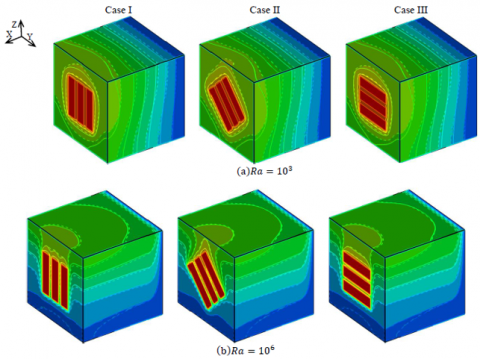Figure 5. Isotherms obtained for $\phi=0$  (_ _ _ ) and $\phi=0.1$ (____) and different Ra numbers

## 6.png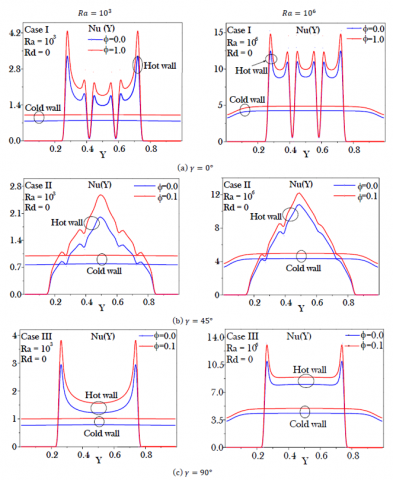Figure 6. Profiles of $N u_{L}(Y)$ on the active walls for different combinations ($R a, \phi$)

It can be also seen that cases I and III exhibit a perfect symmetrical profile about the vertical mid plane. This can be explained by the fact that the heat sources are also symmetrical with respect to the same plane. In case I (Figure 6 (a)), the heat transfer produced by the three elements is well separated. The results show that the central element gives less interesting heat transfer than the two others. The obtained profiles for each element have a parabolic form with a minimum value almost located in its center. For case II, the obtained profiles have a triangular form. The increasing ramp due to a progressive contribution of the elements starts from $Y \sim 0.17 .$ The decreasing one starts from $Y=0.5$ and leads to zero heat transfer beyond $Y \sim 0.82 .$ In case III, the three elements simultaneously contribute to the heat transfer in Z direction. For $R a=10^{3},$ the obtained profile keeps its parabolic shape. By cons, for $R a=10^{6}, N u_{L}(Y)$ remains almost constant over the interval $0.25 \leq Y \leq 0.75$ occupied by the heated parts. On the cold wall of the cavity, so far from the heating elements, $N u_{L}(Y)$ is independent of the studied case. For $R a=10^{3},$ the obtained lines are perfectly horizontal $\left(N u_{L}(Y)=N u\right) .$ This shows a weak three-dimensional aspect which apparently only manifests itself near the uppmer corners of the cavity (Figure 4 ). For $R a=$ $10^{6},$ a drop of about $20 \%$ in the local heat transfer is recorded near the vertical passive walls ( $Y$ close to 0 or 1 ). Indeed, the passive walls (horizontal and vertical) tend to slow down the circulation of the fluid which plays the main role on heat exchange in the cavity for high $R a$ numbers.

The radiation effect on the flow structure of the $C u$ -water nanofluid is illustrated by presenting in Figure $7,$ the standardized velocity $W / W_{0 \max }$ and the temperature profiles along the centerline $Y=Z=0.5$ for $\gamma=45^{\circ}$ and different combinations of parameters $(R a, \phi, R d) . W_{0 \max }$ is the reference maximal velocity corresponding to $\phi=R d=0$ For $R a=10^{3},$ it can be seen that on the horizontal line $Y=$ $Z=0.5,$ the nanofluid temperature is independent of the parameters $\phi$ and $R d .$ Moreover, only the volume fraction of the nanoparticles seems to have an effect on the vertical velocity component $W$. By switching to the high values of $R a$ numbers, the effects of the parameters $\phi$ and $R d$ are more noticeable. By increasing $\phi$ then $R d$, the boundary layers on both active walls become thicker. The obtained maximum velocity in the presence of radiation is significantly higher. This is because the presence of radiation would increase the overall temperature of the nanofluid in the vicinity of the heated wall, consequently, higher buoyancy force. In other words, nanoparticles and radiation increase nanofluid flow rate in the cavity. Note also that the nanofluid velocities and the temperature gradients are greater at the hot wall which indicates that the local Nusselt number is higher on the heating elements than on the cold wall. Indeed, on the fully active wall, the heat exchanges are carried out on a larger surface and should, therefore, have reduced values. From the temperature profiles, it also interesting to note that the presence of the radiation and the nanoparticles effects tend to reduce the temperature gradients near the active walls.

## 7.png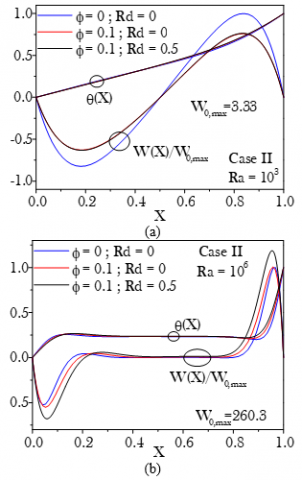Figure 7. Profiles of $W / W_{0 \max }$ and $\theta$ on the line $Y=Z=0.5$ for different combinations $(R a, \phi, R d)$

4.4 Average heat transfer

In order to clarify the heat transfer enhancement throughout the cavity for different investigated cases, the variation of the the cavity for different investigated cases, the variation of the average Nusselt number as a function of $R a, \phi$ and $R d$ are illustrated in Figure 8 (a-c). According to the results of Figure $8(\mathrm{a}),$ giving the average heat transfer as a function of Rayleigh number for different inclinations $\gamma,$ no effect of the three heating elements orientation on the heat transfer rate was detected for three sets of parameters $\phi$ and $R d: R d=\phi=0$; $R d=0$ and $\phi=0.1 ; R d=0.5$ and $\phi=0.1 .$ Thus, thereafter, the inclination angle effect can be ignored and the study can be continued by limiting itself to a single case: $\gamma=90^{\circ}$ for example. However, from Figure 8 (a), it is also clear that the average Nusselt number increases with the three parameters $R a, \phi$ and $R d .$ Indeed, for $R a=10^{6},$ a $14 \%$ improvement in convective heat transfer was obtained by adding only a fraction of 10% of the Cu nanoparticles. A further 34% increase was then obtained when the thermal radiation is taken into account. The only effect of the volume fraction $\phi$ (the radiation is not taken into account on the mean convective heat transfer is illustrated at different $R a$ numbers in Figure 8 (b). The average Nusselt number is found as an increasing function of the Rayleigh number and nanoparticule volume fraction due to the dominance of the convective regime and the improvement of nanofluid thermal conductivity. Similar trend could also be seen from Figure 8 (c) showing the effect of the $R d$ parameter for $\phi=0.1$ and different values of $R a$ number.. Indeed, the presence of the thermal radiation increases the effective thermal conductivity of the nanofluid. Hence, the heat could be easily transferred from the hot wall into the cavity and vice versa on the cold one.

## 8.png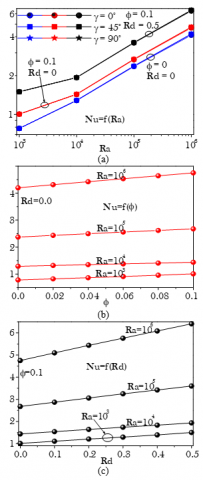Figure 8. Evolution of the Nusselt number according to the parameters $R a, \gamma, \phi$ and $R d$

Figures $9(a-c)$ illustrate for $\phi=0.1$ and $R d=0.5,$ the effects of the type of the nanoparticles $\left(A g, A l_{2} O_{3}, C u, T i O_{2}\right)$ as well as that of certain geometrical parameters of the heating elements, such as their common aspect ratio $A$ and the distance $D$ separating their centers. As it can be seen, the variation of these parameters induces a significant change in the overall (convective and radiative) heat transfer rate within the cavity. Figure 9 (a) shows that the nanoparticles of $C u$ and $A g$ lead to the highest heat transfer rate over the whole range of the Rayleigh number. In addition, titanium nanoparticles lead to the lowest heat transfer rate. The obtained differences caused by the change of the nanoparticles do not exceed 3% and can be attributed simply to their thermal conductivities which have quite high values for $C u$ and $A g$ nanoparticles. From the results of Figure 9 (b), there is an optimum distance $D \approx 0.28$ between the three elements to obtain the highest heat transfer. This is due to two antagonistic effects: the upper heated element which releases a decreasing amount of heat as it approaches the upper passive wall and the lower one releasing more energy if it approaches the bottom wall. The differences recorded when changing the distance $D$ can be significant and amount to $18 \% .$ Figure 9 (c) illustrates the evolution of the average Nusselt number according to the heating element aspect ratio $A .$ The distance $D$ is set to the found optimum value $D=0.28 .$ The obtained results show that it is possible to improve the overall heat transfer by increasing the aspect ratio of the isothermal elements. An improvement in heat transfer of $16.3 \%$ is obtained by passing from $A=2.25$ to $A=6.25 .$ Indeed, by decreasing the ratio $A,$ the amount of nanofluid circulating in the cavity without interacting with the heating components increases.

## 9.png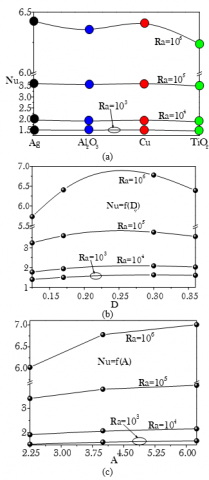Figure 9. Evolution of the Nusselt number as a function of type of nanoparticles (a), spacing D  (b) and aspect ratio (c) of the heating elements for different Rayleigh numbers

5. Conclusions

The finite volume method is used to analyze the effect due to the orientation of three heating elements on the flow structure and the heat transfer of several nanofluids in a partially heated cubical cavity. In this study the thermal radiation often ignored in previous studies is taken into consideration and it was treated based on the Rosseland approximation commonly used for optically thick media. The main results of the numerical analysis are summarized as follows:

Although the inclination angle $\gamma$ of the elements significantly changes the thermal and the local heat transfer behaviors in the vicinity of the hot wall, its effect on the average Nusselt number has not been detected.

The influence of the third direction has been demonstrated through the isotherms in the vicinity of the heated wall, the streamlines inside the cavity as well as the local Nusselt number on the cold surface.

The thermal radiation contributes to a widening of the boundary layers and reduces the temperature gradients near the active walls of the cavity.

The boundary layers are seen to be thicker on the partially active wall and wider on the fully active one.

The heat transfer increases with increasing the Rayleigh number, the volume fraction of nanoparticles, the radiation parameter and the aspect ratio of the heated elements.

According to the heated elements spacing D, optimum heat transfer occurred for $D \approx 0.28$.

The Cu and Ag nanoparticles ensure high heat transfer phenomena than those of Al2O3 and TiO2.

Nomenclature
 A aspect ratio of elements D distance between elements g gravitational acceleration, m.s-2 L,H,W dimensions of the cubical cavity, m k thermal conductivity, W/mK Nu Nusselt number P pressure, Pa Pr Prandtl number Ra Rayleigh number Rd radiation parameter T temperature, K U,V,W dimensionless Cartesian velocities x,y,x Cartesian coordinates, M X,Y,Z dimensionless Cartesian coordinates Greek symbols $\alpha$ thermal diffusivity, m2. s-1 $\beta$ thermal expansion coefficient, K-1 $\beta_{\mathrm{R}}$ mean absorption coefficient, m-1 $\gamma$ angle of inclination, ° $\delta$ Kronecker symbol $\Delta \mathrm{T}$ temperature difference, K $\theta$ dimensionless temperature $v$ kinematic viscosity, m2/s $\rho$ density, Kg/m3 $\sigma$ Stefan Boltzmann constant, W/K4m2 $\tau$ dimensionless time $\phi$ solid volume fraction Subscripts C Convection f fluid (pure water) H hot L local Max maximum nf nanofluid s solid
References

 Choi, U.S., Eastman, J.A. (1995). Enhancing thermal conductivity of fluids with nanoparticles. ASME Fluids Engineering Division, 231: 99-105.

 Haddad, Z., Oztop, H.F., Abu-Nada, E., Mataoui, A. (2012). A review on natural convective heat transfer of nanofluids. Renewable and Sustainable Energy Reviews, 16(7): 5363-5378. https://doi.org/10.1016/j.rser.2012.04.003

 Oztop, H.F., Abu-Nada, E. (2008). Numerical study of natural convection in partially heated rectangular enclosures filled with nanofluids. International Journal of Heat and Fluid Flow, 29(5): 1326-1336. https://doi.org/10.1016/j.ijheatfluidflow.2008.04.009

 Hosseini, M., Mustafa, M.T., Jafaryar, M., Mohammadian, E. (2014). Nanofluid in tilted cavity with partially heated walls. Journal of Molecular Liquids, 199: 545-551. https://doi.org/10.1016/j.molliq.2014.09.051

 Bhuiyanaa, H., Alamb, M.S., Alim, M.A. (2017). Natural convection of water-based nanofluids in a square cavity with partially heated of the bottom wall. Procedia Engineering, 194: 435-441. https://doi.org/10.1016/j.proeng.2017.08.168

 Boudjeniba1, B., Laouer, A., Laouar, S., Mezaache, E. (2019). Transition to chaotic natural convection of Cu-water nanofluid in an inclined square enclosure. International Journal of Heat and Technology, 37(2): 413-422. https://doi.org/10.18280/ijht.370206

 Cho, C.C. (2014). Heat transfer and entropy generation of natural convection in nanofluid-filled square cavity with partially-heated wavy surface. International Journal of Heat and Mass Transfer, 77: 818-827. https://doi.org/10.1016/j.ijheatmasstransfer.2014.05.063

 Sheremet, M.A., Cimpean, D.S., Pop, I. (2017). Free convection in a partially heated wavy porous cavity filled with a nanofluid under the effects of Brownian diffusion and thermophoresis. Applied Thermal Engineering, 113: 413-418. https://doi.org/10.1016/j.applthermaleng.2016.11.033

 Sheremet, M.A., Oztop, H.F., Pop, I. (2016). MHD natural convection in an inclined wavy cavity with corner heater filled with a nanofluid. Journal of Magnetism and Magnetic Materials, 416: 37-47. https://doi.org/10.1016/j.jmmm.2016.04.061

 Mansour, M.A., Siddiq, S., Gorla, R.S.R., Rashad, A.M. (2018). Effects of heat source and sink on entropy generation and MHD natural convection of a Al2O3-Cu/water hybrid nanofluid filled with square porous cavity. Thermal Science and Engineering Progress, 6: 57-71. https://doi.org/10.1016/j.tsep.2017.10.014

 Wang, L., Shi, B., Chai, Z. (2017). Effects of temperature-dependent properties on natural convection of nanofluids in a partially heated cubic enclosure. Applied Thermal Engineering, 128: 204-213. https://doi.org/10.1016/j.applthermaleng.2017.09.006

 Ul Haq, R., Soomro, F.A., Hammouch, Z., Rehman, S.U. (2018). Heat exchange within the partially heated C-shape cavity filled with the water based SWCNTs. International Journal of Heat and Mass Transfer, 127: 506-514. https://doi.org/10.1016/j.ijheatmasstransfer.2018.07.101

 Sheremet, M.A., Pop, I. (2018). Effect of local heater size and position on natural convection in a tilted nanofluid porous cavity using LTNE and Buongiorno's models. Journal of Molecular Liquids, 266: 19-28. https://doi.org/10.1016/j.molliq.2018.06.065

 Guestal, M., Kadja, M., Hoang, T. (2018). Study of heat transfer by natural convection of nanofluids in a partially heated cylindrical enclosure. Case Studies in Thermal Engineering, 11: 135-144. https://doi.org/10.1016/j.csite.2018.01.008

 Ul Haq, R., Aman, S. (2019). Water functionalized CuO nanoparticles filled in a partially heated trapezoidal cavity with inner heated obstacle: FEM approach. International Journal of Heat and Mass Transfer, 128: 401-417. https://doi.org/10.1016/j.ijheatmasstransfer.2018.08.088

 Wang, L., Yang, X., Huang, C., Chai, Z., Shi, B. (2019). Hybrid lattice Boltzmann-TVD simulation of natural convection of nanofluids in a partially heated square cavity using Buongiorno’s model. Applied Thermal Engineering, 146: 318-327. https://doi.org/10.1016/j.applthermaleng.2018.09.109

 Selimefendigil, F., Öztop, H.F. (2018). Role of magnetic field and surface corrugation on natural convection in a nanofluid filled 3D trapezoidal cavity. International Communications in Heat and Mass Transfer, 95: 182-196. https://doi.org/10.1016/j.icheatmasstransfer.2018.05.006

 Jain, S., Gupta, P. (2019). Entropy generation analysis of carbon nanotubes nanofluid 3D flow along a nonlinear inclined stretching sheet through porous media. International Journal of Heat and Technology, 37(1): 131-138. https://doi.org/10.18280/ijht.370116

 Aun, T.S., Abdullah, M.Z., Gunnasegaran, P. (2017). Influence of low concentration of diamond water nanofluid in loop heat pipe. International Journal of Heat and Technology, 35(3): 539-548. https://doi.org/10.18280/ijht.350310

 Matyska, C., Moser, J., Yuen, D.A. (1994). The potential influence of radiative heat transfer on the formation of megaplumes in the lower mantle. Earth and Planetary Science Letters, 125(1-4): 255-266. https://doi.org/10.1016/0012-821x(94)90219-4

 Rosenberger, F., Feigelson, R.S. (1981). Fundamentals of crystal growth I: Macroscopic equilibrium and transport concepts. Physics Today, 34(2): 77-78. https://doi.org/10.1063/1.2914446

 Gardon, R. (1961). A review of radiant heat transfer in glass. Journal of the American Ceramic Society, 44(7): 305-312. https://doi.org/10.1111/j.1151-2916.1961.tb15914.x

 Karimipour, A. (2017). A novel case study for thermal radiation through a nanofluid as a semitransparent medium via discrete ordinates method to consider the absorption and scattering of nanoparticles along the radiation beams coupled with natural convection. International Communications in Heat and Mass Transfer, 87: 256-269. https://doi.org/10.1016/j.icheatmasstransfer.2017.07.020

 Ghalambaz, M., Sabour, M., Pop, I. (2016). Free convection in a square cavity filled by a porous medium saturated by a nanofluid: Viscous dissipation and radiation effects. Engineering Science and Technology, an International Journal, 19(3): 1244-1253. https://doi.org/10.1016/j.jestch.2016.02.006

 Raptis, A., Perdikis, C., Takhar, H.S. (2004). Effect of thermal radiation on MHD flow. Applied Mathematics Computation, 153(3): 645-649. https://doi.org/10.1016/s0096-3003(03)00657-x

 Mahmoud, M.A.A. (2007). Thermal radiation effects on MHD flow of a micropolar fluid over a stretching surface with variable thermal conductivity. Physica A: Statistical Mechanics and its Applications, 375(11): 401-410. https://doi.org/10.1016/j.physa.2007.12.001

 Ravnik, J., Skerget, L., Hribersek, M. (2010). Analysis of three-dimensional natural convection of nanofluids by BEM. Engineering Analysis with Boundary Elements, 34(12): 1018-1030. https://doi.org/10.1016/j.enganabound.2010.06.019